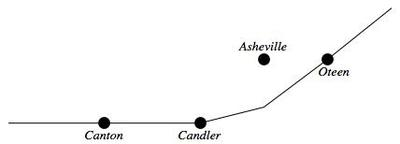# Map distance

Alignments to Content Standards: 7.G.A.1

On the map below, $\frac14$ inch represents one mile. Candler, Canton, and Oteen are three cities on the map.1. If the distance between the real towns of Candler and Canton is 9 miles, how far apart are Candler and Canton on the map?

2. If Candler and Oteen are $3\frac12$ inches apart on the map, what is the actual distance between Candler and Oteen in miles?

## IM Commentary

The purpose of this task is for students to translate between information provided on a map that is drawn to scale and the distance between two cities represented on the map. This task is a very straightforward application of the mathematics described in 7.G.A.1. This task would be appropriate for assessment.

## Solution

1. A distance of nine miles means 9 quarter inches on the map. This is $$9\times\frac14 = \frac94$$ or $2\frac14$ inches between Candler and Canton on the map.2. How many $\frac14$ inches are in $3\frac12$ inches? To find this, we divide: $$3\frac12 \div \frac14 = \frac72 \times \frac41 = 14$$ So it is 14 miles between Chandler and Oteen.